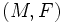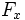Deicke's theorem

Let$(M,F)$ be a Finsler manifold. Let$x \in M$ be a point. The norm$F_x$ in the tangent space at$x$ is a Euclidean norm if and only if the mean Cartan torsion is 0 for all tangent vectors at$x$.